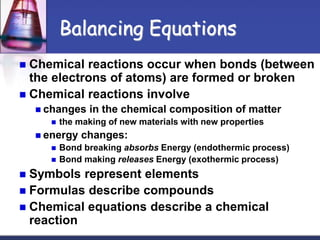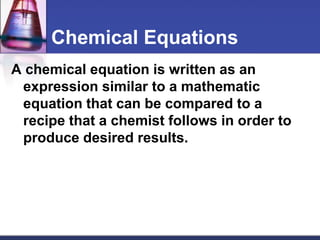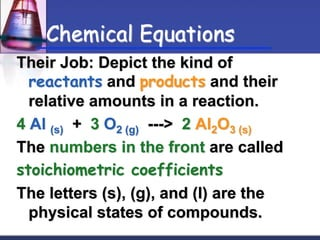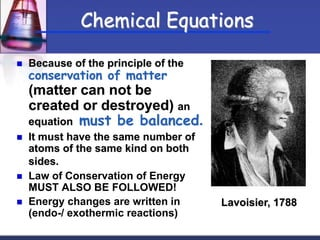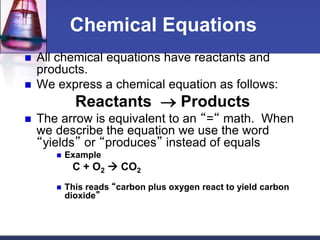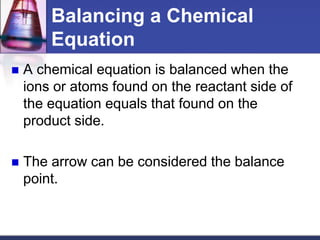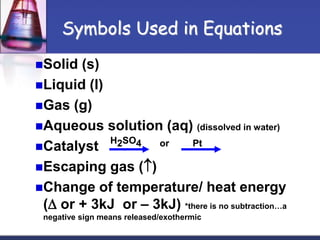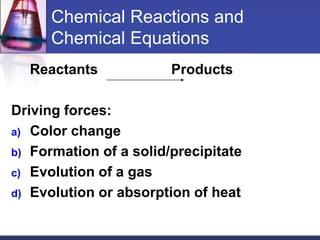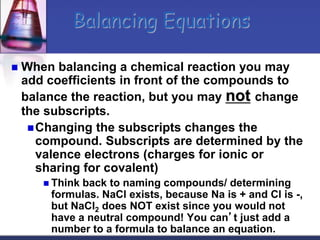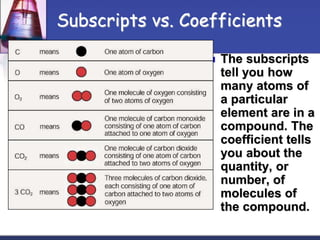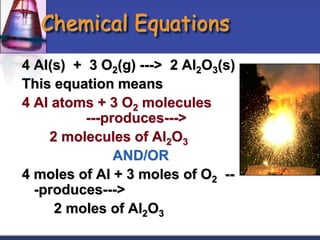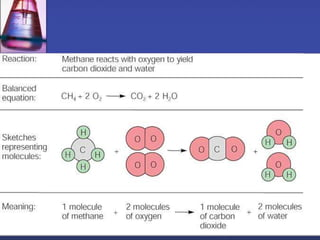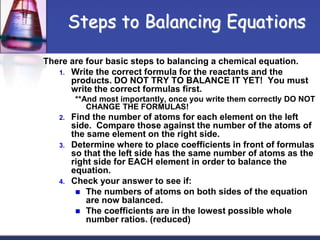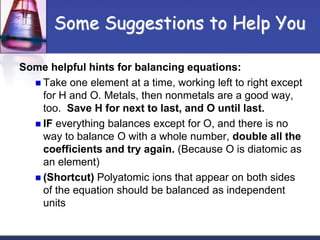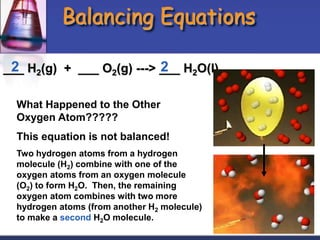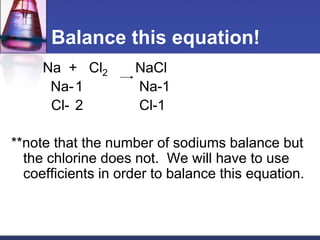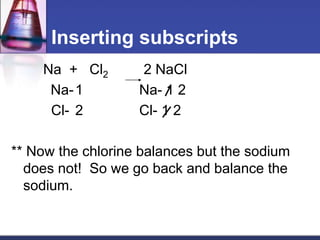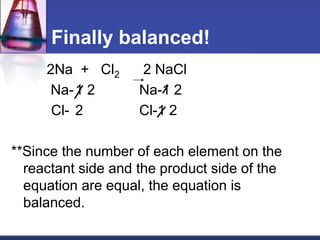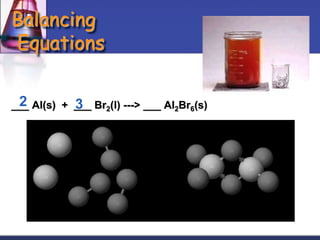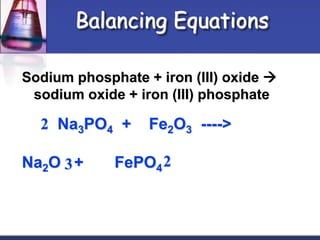1 de 20

### balancing equations.ppt

1.  Chemical reactions occur when bonds (between the electrons of atoms) are formed or broken  Chemical reactions involve  changes in the chemical composition of matter  the making of new materials with new properties  energy changes:  Bond breaking absorbs Energy (endothermic process)  Bond making releases Energy (exothermic process)  Symbols represent elements  Formulas describe compounds  Chemical equations describe a chemical reaction Balancing Equations
2. Chemical Equations A chemical equation is written as an expression similar to a mathematic equation that can be compared to a recipe that a chemist follows in order to produce desired results.
3. Chemical Equations Their Job: Depict the kind of reactants and products and their relative amounts in a reaction. 4 Al (s) + 3 O2 (g) ---> 2 Al2O3 (s) The numbers in the front are called stoichiometric coefficients The letters (s), (g), and (l) are the physical states of compounds.
4.  Because of the principle of the conservation of matter (matter can not be created or destroyed) an equation must be balanced.  It must have the same number of atoms of the same kind on both sides.  Law of Conservation of Energy MUST ALSO BE FOLLOWED!  Energy changes are written in (endo-/ exothermic reactions) Lavoisier, 1788 Chemical Equations
5. Chemical Equations  All chemical equations have reactants and products.  We express a chemical equation as follows: Reactants  Products  The arrow is equivalent to an “=“ math. When we describe the equation we use the word “yields” or “produces” instead of equals  Example C + O2  CO2  This reads “carbon plus oxygen react to yield carbon dioxide”
6. Balancing a Chemical Equation  A chemical equation is balanced when the ions or atoms found on the reactant side of the equation equals that found on the product side.  The arrow can be considered the balance point.
7. Solid (s) Liquid (l) Gas (g) Aqueous solution (aq) (dissolved in water) Catalyst H2SO4 or Pt Escaping gas () Change of temperature/ heat energy ( or + 3kJ or – 3kJ) *there is no subtraction…a negative sign means released/exothermic Symbols Used in Equations
8. Chemical Reactions and Chemical Equations Reactants Products Driving forces: a) Color change b) Formation of a solid/precipitate c) Evolution of a gas d) Evolution or absorption of heat
9.  When balancing a chemical reaction you may add coefficients in front of the compounds to balance the reaction, but you may not change the subscripts. Changing the subscripts changes the compound. Subscripts are determined by the valence electrons (charges for ionic or sharing for covalent)  Think back to naming compounds/ determining formulas. NaCl exists, because Na is + and Cl is -, but NaCl2 does NOT exist since you would not have a neutral compound! You can’t just add a number to a formula to balance an equation. Balancing Equations
10. Subscripts vs. Coefficients  The subscripts tell you how many atoms of a particular element are in a compound. The coefficient tells you about the quantity, or number, of molecules of the compound.
11. Chemical Equations 4 Al(s) + 3 O2(g) ---> 2 Al2O3(s) This equation means 4 Al atoms + 3 O2 molecules ---produces---> 2 molecules of Al2O3 AND/OR 4 moles of Al + 3 moles of O2 -- -produces---> 2 moles of Al2O3
12. There are four basic steps to balancing a chemical equation. 1. Write the correct formula for the reactants and the products. DO NOT TRY TO BALANCE IT YET! You must write the correct formulas first. **And most importantly, once you write them correctly DO NOT CHANGE THE FORMULAS! 2. Find the number of atoms for each element on the left side. Compare those against the number of the atoms of the same element on the right side. 3. Determine where to place coefficients in front of formulas so that the left side has the same number of atoms as the right side for EACH element in order to balance the equation. 4. Check your answer to see if:  The numbers of atoms on both sides of the equation are now balanced.  The coefficients are in the lowest possible whole number ratios. (reduced) Steps to Balancing Equations
13. Some Suggestions to Help You Some helpful hints for balancing equations:  Take one element at a time, working left to right except for H and O. Metals, then nonmetals are a good way, too. Save H for next to last, and O until last.  IF everything balances except for O, and there is no way to balance O with a whole number, double all the coefficients and try again. (Because O is diatomic as an element)  (Shortcut) Polyatomic ions that appear on both sides of the equation should be balanced as independent units
14. Balancing Equations ___ H2(g) + ___ O2(g) ---> ___ H2O(l) 2 2 What Happened to the Other Oxygen Atom????? This equation is not balanced! Two hydrogen atoms from a hydrogen molecule (H2) combine with one of the oxygen atoms from an oxygen molecule (O2) to form H2O. Then, the remaining oxygen atom combines with two more hydrogen atoms (from another H2 molecule) to make a second H2O molecule.
15. Balance this equation! Na + Cl2 NaCl Na-1 Na-1 Cl- 2 Cl-1 **note that the number of sodiums balance but the chlorine does not. We will have to use coefficients in order to balance this equation.
16. Inserting subscripts Na + Cl2 2 NaCl Na-1 Na- 1 2 Cl- 2 Cl- 1 2 ** Now the chlorine balances but the sodium does not! So we go back and balance the sodium.
17. Finally balanced! 2Na + Cl2 2 NaCl Na-1 2 Na-1 2 Cl- 2 Cl-1 2 **Since the number of each element on the reactant side and the product side of the equation are equal, the equation is balanced.
18. Balancing Equations ___ Al(s) + ___ Br2(l) ---> ___ Al2Br6(s) 2 3
19. Balancing Equations Sodium phosphate + iron (III) oxide  sodium oxide + iron (III) phosphate Na3PO4 + Fe2O3 ----> Na2O + FePO42 2 3